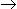# Aptitude Test-Problems of Numbers Questions(Mcq)

Numbers Aptitude Test Questions(Mcq)

All Questions Answers are Given Below Check it

Question 1.The Unit Digit in the Produt (784×618×917×463)
A)2
B)3
C)5
D)4

Question 2. 9587-X=7429-4358 then X=?
A)5011
B)6516
C)6511
D)6173

Question 3.(796×796-204×204)=?
A)592000
B)592001
C)582762
D)783623

Question 4.(87×87+61×61-2×87×61)=?
A)295
B)562
C)195
D)196

Question 5. What is the Unit Digit of  7105   ?
A)1
B)5
C)7
D)9

 Question 6.Which one of the following is not a prime number?
A)31
B)61
C)91
D)87

 Question 7.(112 x 54) = ?
A)77000
B)70000
C)82444
D)77733
 Question 8.What least number must be added to 1056, so that the sum is completely divisible by 23 ?
A)3
B)5
C)6
D)2

Question 91397 x 1397 = ?
A)1951609.
B)1951611
C)1826351
D)3682251

Question 10How many of the following numbers are divisible by 132 ?
264, 396, 462, 792, 968, 2178, 5184, 6336
A)8
B)4
C)6
D)5
Question 11If 6 + 12 + 18 + 24 + --- = 1800, then find the number of terms in the series.
A)24
B)
C)
D)21
Question 121 + 2 + 3 + ---- 50 = ?
A)1276
B)1271
C)1275
D)1280
Question 13.Find the solution of(450 + 280)2 – (450 – 280) 2/(450 × 280)

B)4

D)5
Question 14The remainder is 3, when a number is divided 5. If the square of this number is divided by 5, then what is  remainder?

D)4
Question 15Find the number of terms in geometric progression 3, 6, 12, 24, ---- , 384.
A)8
B)7
C)5
D)6

Question 1: The correct answer is the
Unit Digit in the given product=Unit Digit in(4×8×7×3)=2

Question 2: The correct answer is

9587-X=7429-4358 then X=?
then 9887-X=3071
X=9587-3071=6516

Question 3: The correct answer is
By Using this formula-(a²-b²)=(a+b)(a-b)
796×796-204×204=(796)²-(204)²
=(796+204)(796-204)=1000×593=592000

Question 4: The correct answer is
By Using the fomula-(a²+b²-2ab)=(a-b)²
where a=87,b=61
(87²+61²-2×81×61)=(87-61)²=(26)²=(20+6)²
=20²+6²-2x20x6=(400+36-240)
=(436-240)=196.

Question 5: The correct answer is
the Unit Digit of  7105 =Unit Digit in [(74)26×7]
The Unit Digit in  (74)26=1
then Unit Digit in  7105 =(1x7)=7

Question 6: The correct answer is
91 is divisible by 7. So, it is not a prime number

Question 7: The correct answer is
(112 x 54) = 112 x10/24=112 x 104/16=1120000/6= 70000

Question 8: The correct answer is
``` 23) 1056 (45
92
---
136
115
---
21
---

Required number = (23 - 21)
= 2.```
`   `
Question 9: The correct answer is

 1397 x 1397 = (1397)2 = (1400 - 3)2 = (1400)2 + (3)2 - (2 x 1400 x 3) = 1960000 + 9 - 8400 = 1960009 - 8400 = 1951609.
Question 10: The correct answer is
132 = 4 x 3 x 11
So, if the number divisible by all the three number 4, 3 and 11, then the number is divisible by 132 also.
26411,3,4 (/)
39611,3,4 (/)
46211,3 (X)
79211,3,4 (/)
96811,4 (X)
217811,3 (X)
51843,4 (X)
633611,3,4 (/)
Therefore the following numbers are divisible by 132 : 264, 396, 792 and 6336.
Required number of number = 4.

Question 11: The correct answer is
This is an Arithmetic Progression, in which x = 6, y = 6, sum of terms = 1800
 Sum of n terms = n [2x + (n – 1)y] 2
Substituting the given values, we get
 1800 = n [2 × 6 + (n – 1)6] 2

Solving we get,
1800 = 3n (n + 1)
n(n +1) = 600
n2 + n = 600
25 × 24 = 600
Therefore,
n2 + 25n – 24n – 600 = 0
(n + 25) (n – 24) = 0
24 and -25 are the two solutions obtained. Only positive value can be considered. Hence, n = 24
The number of terms in the series = 24.

Question 12: The correct answer is
This is an Arithmetic Progression, here a = 1, last term = 50 and n = 50
 Sum of n terms = n (a + l) 2

Substituting the given values, we get

 Sum of n terms = 50 (1 + 50) 2
Sum of n terms = 25 × 51 = 1275

Question 13: The correct answer is
By Using Formula
(a + b)2 = a2 + 2ab + b2
(a – b)2 = a2 – 2ab + b2
If we substitute these formulae, we get
 (a + b)2 – (a – b)2 = (a2 + 2ab + b2 – a2 + 2ab – b2) = 4ab = 4 (a × b) (a × b) ab

Question 14: The correct answer is

Dividend = [(Divisor × Quotient)] + Remainder
It is given that, the remainder is 3, when a number (dividend) is divided 5(divisor).
Dividend and quotient are unknown, hence assume dividend as X and quotient as Y.
X = (5Y) + 3
The square of this number is divided by 5, therefore
X2 = (5Y + 3)2
Simplifying we get,
X2 = (25Y2 + 30Y + 9)
On dividing this equation, we get
5(5Y2 + 6Y + 1) + 4
The remainder is 4, because 9 is not exactly divisible by 5 and we get 4 as remainder

Question 15: The correct answer is

 Here, x = 3, r = 6 = 2 3
We have to find the number of terms
Nth term = 384
nth term = xr (n -1)
384 = 3 x 2 (n -1)
 2(n – 1) = 384 = 128 3
2 (n -1) = 128
27 = 128

Therefore,
2 (n -1) = 27
(n – 1) = 7
n = 7 + 1 = 8

Hence, number of terms = 8